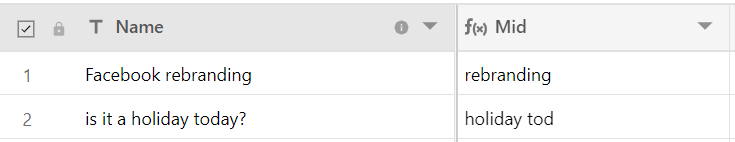In this article, we will learn how you can extract text from a

1. LEN ()

LEN returns the length of a string. You can reference a value/column/string within the function following this format: LEN({COLUMN NAME})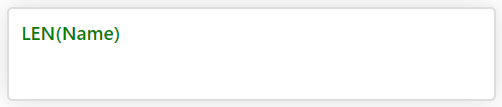Result: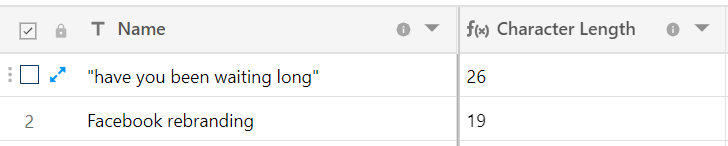Some of the interesting ways you can use LEN () function

Displaying a message based on character count

Here, we can use IF statements to know whether characters are under 35 limit and output a message depending on the character count. Whether you want to count characters in your tweet or managing your SEO title & meta descriptions, you can use the function below: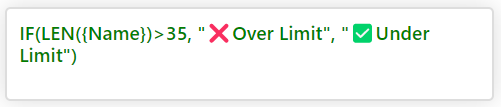Result: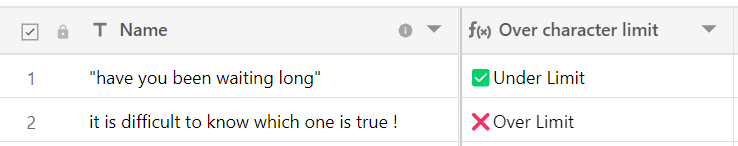2. Counting words

You can use a similar formula to count the number of spaces in a text field, which in turn will give you a total count of words.

Formula: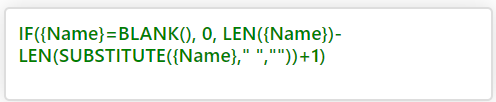Result: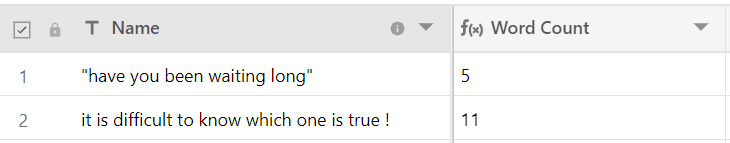3. LEFT() and RIGHT()

The LEFT() and RIGHT() functions work similarly to one another by extracting a certain number of characters from the beginning (left) or end (right) of a string.

These are helpful functions to use when you need to extract data from a number of rows that share a similar structure.

Syntax: LEFT(String, howMany)
Example: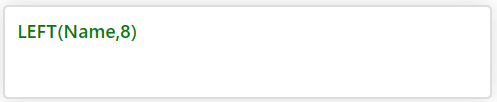Result: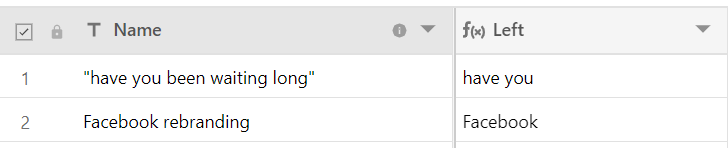## 4. MID()This function lets you extract a certain number of characters starting at a specific place within a string.

Syntax: MID(String, wheretostart, count)

Example: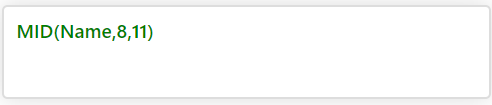Result: